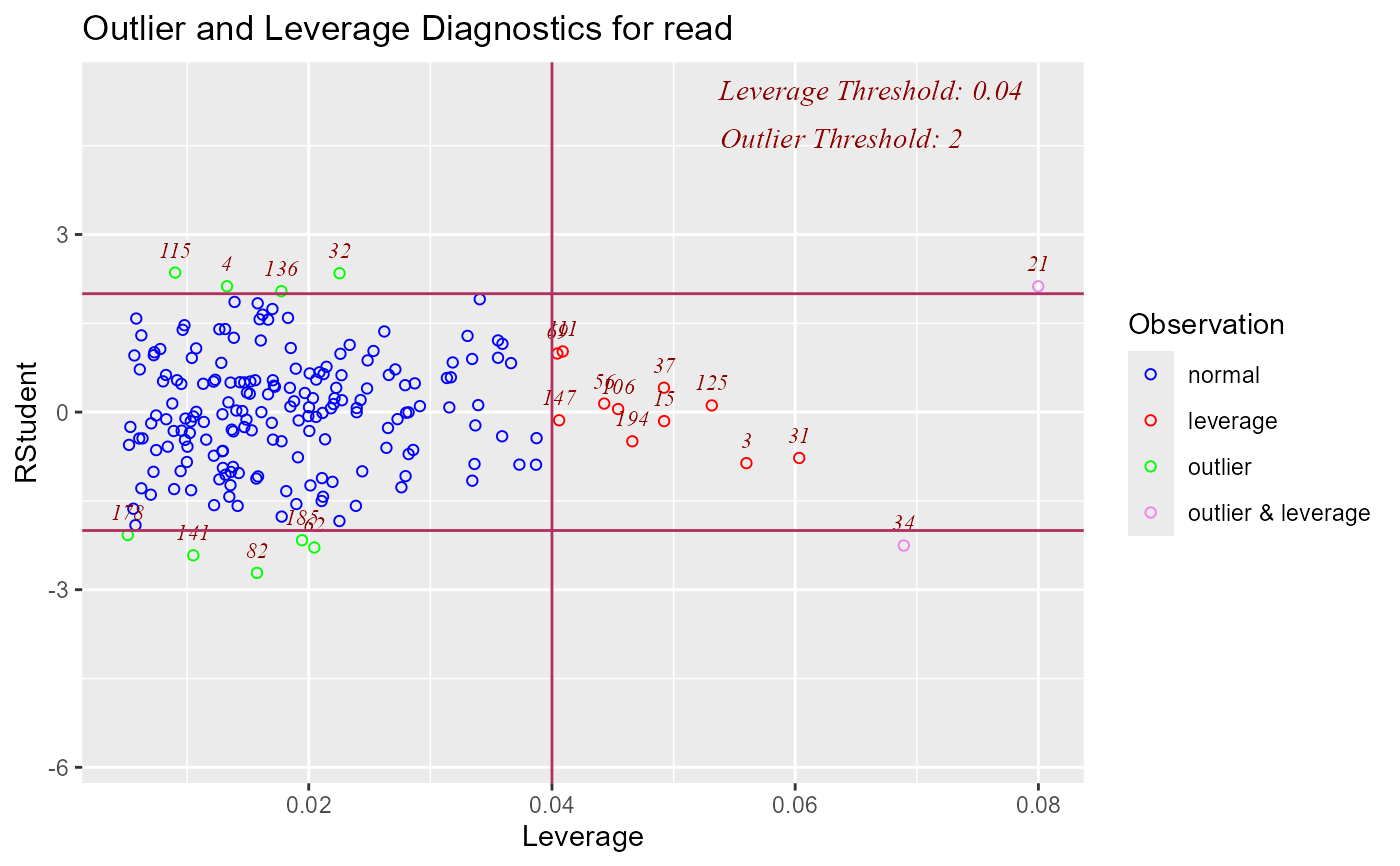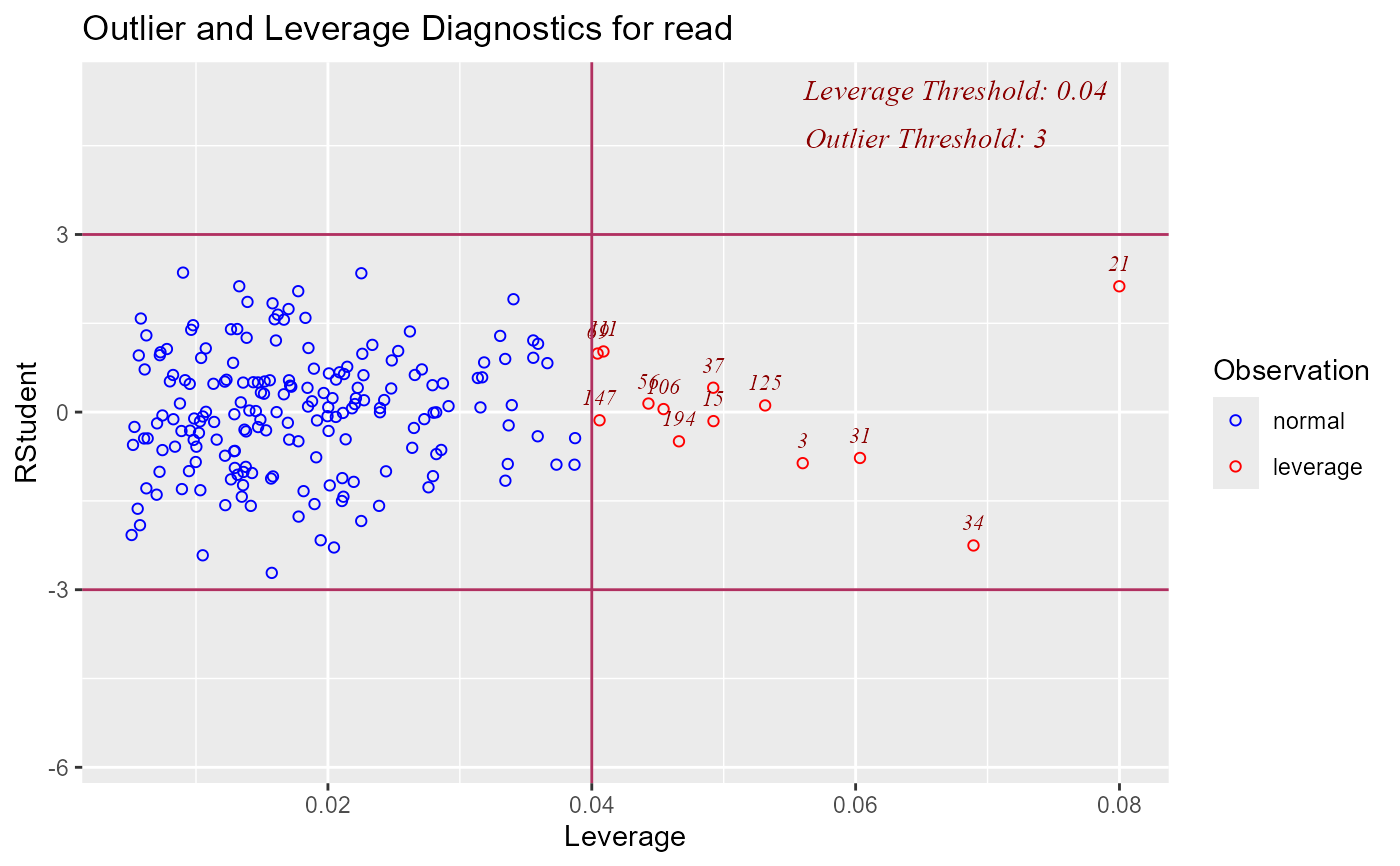Graph for detecting outliers and/or observations with high leverage.

## Usage

ols_plot_resid_lev(model, threshold = NULL, print_plot = TRUE)

## Arguments

model

An object of class lm.

threshold

Threshold for detecting outliers. Default is 2.

print_plot

logical; if TRUE, prints the plot else returns a plot object.

model <- lm(read ~ write + math + science, data = hsb)